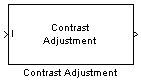Adjust image contrast using linear scaling

•Libraries:
Computer Vision Toolbox / Analysis & Enhancement

## Description

The Contrast Adjustment block adjusts the contrast of an image by linearly scaling the pixel values between specified upper and lower limits. Pixel values that are above or below the specified range are saturated to the upper or lower limit value, respectively. For more information, see Adjust Contrast of Image.

## Ports

### Input

expand all

Input image, specified as an M-by-N matrix or an M-by-N-by-C array, where C is the number of color channels in the input image.

Data Types: `single` | `double` | `int8` | `int16` | `uint8` | `uint16` | `Boolean` | `fixed point`

### Output

expand all

Output image, returned as an M-by-N matrix or an M-by-N-by-C array, where C is the number of color channels.

Data Types: `single` | `double` | `int8` | `int16` | `uint8` | `uint16` | `Boolean` | `fixed point`

## Parameters

expand all

Specify how the block determines the pixel value range of the input image as one of these options:

• `Full input data range [min max]` — Range set to the minimum and maximum pixel values in the input image.

• `User-defined range` — Select this value to enable a Range [low high] parameter. Specify the Range [low high] parameter as a two-element vector of nonnegative values, in which the first element is the lower limit of the input pixel value range and the second element is the upper limit of the input pixel value range.

• `Range determined by saturating outlier pixels` — Range specified by saturating the outlier pixels. The block determines the range based on the Percentage of pixels to saturate [low high], Specify number of histogram bins (used to calculate the range when outliers are eliminated), and Number of histogram bins parameters. For more information, see Determine Contrast Adjustment Range by Saturating Outlier Pixels.

Specify the input pixel value range as a two-element vector of nonnegative numbers. The first and second elements of the vector specify the lower and upper limit of the input pixel value range, respectively.

#### Dependencies

To enable this parameter, set the Adjust pixel values from parameter to `User-defined`.

Specify the maximum percentages of pixels to saturate as a two-element vector of nonnegative numbers. Each element must be in the range 0 to 100. The block calculates the lower limit of the input pixel value range such that the percentage of pixels in the input image with values less than the lower limit is at most the value of the first element. Similarly, the block calculates the upper limit of the input pixel value range such that the percentage of pixels in the input image with values greater than the upper limit is at most the value of the second element.

#### Dependencies

To enable this parameter, set the Adjust pixel values from parameter to ```Range determined by saturating outlier pixels```.

Select this parameter to enable the Number of histogram bins parameter, which enables you to specify a custom number of histogram bins for the block to use to calculate the input pixel value range after outliers are eliminated.

#### Dependencies

To enable this parameter, set the Adjust pixel values from parameter to ```Range determined by saturating outlier pixels```.

Specify the number of histogram bins to use to calculate the range for scaling the input pixel values.

#### Dependencies

To enable this parameter, set the Adjust pixel values from parameter to `Range determined by saturating outlier pixels`, and select the Specify number of histogram bins (used to calculate the range when outliers are eliminated) parameter.

Specify how the block determines the pixel value range of the output image as one of these options:

• `Full data type range` — Specifies the output range of pixel values as from the minimum to the maximum value of the input data type.

• `User-defined range` — Select this value to enable a Range [low high] parameter. Specify the Range [low high] parameter as a two-element vector of nonnegative values, in which the first element is the lower limit of the output pixel value range and the second element is the upper limit of the output pixel value range.

Specify the output pixel value range as a two-element vector of nonnegative numbers. The first and second elements of the vector specify the lower and upper limit of the output pixel value range, respectively.

#### Dependencies

To enable this parameter, set the Adjust pixel values to parameter to `User-defined range`.

## Block Characteristics

 Data Types `double` | `fixed point` | `integer` | `single` Multidimensional Signals `no` Variable-Size Signals `yes`

expand all

## Version History

Introduced in R2006b